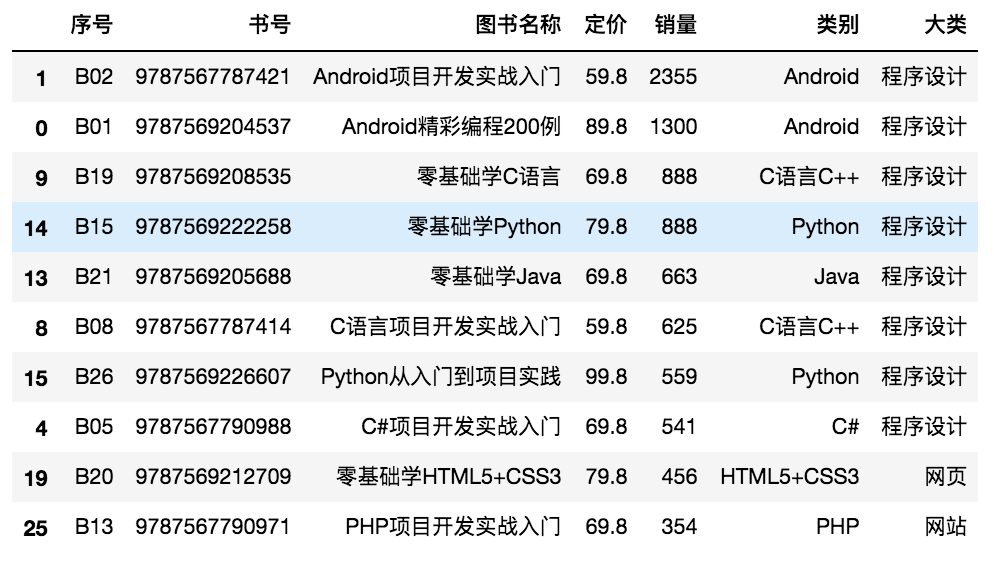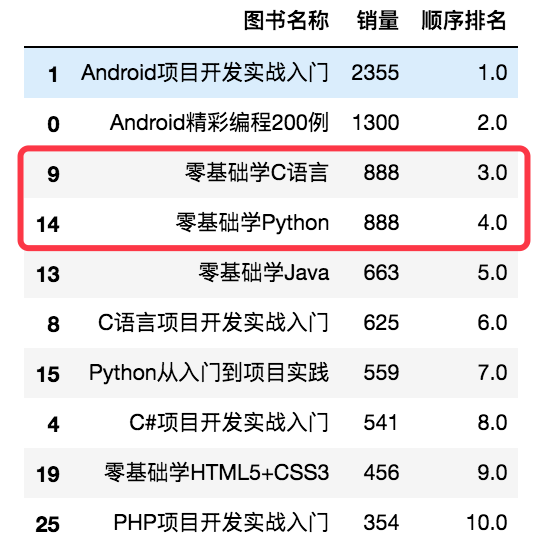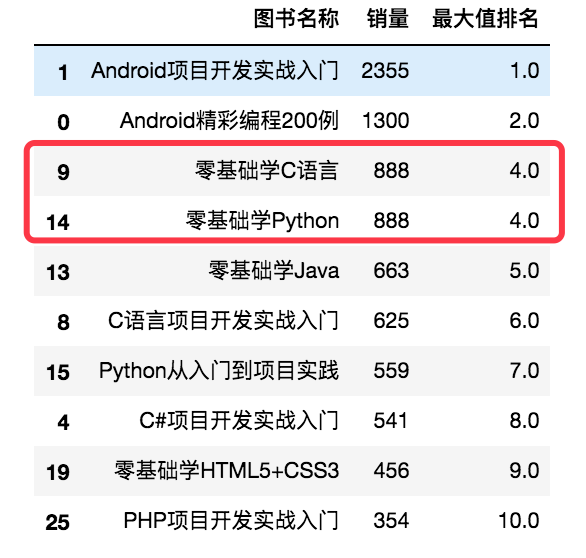数据分析、数据挖掘、可视化是 Python 的众多强项之一，但无论是这几项中的哪一项都必须以数据作为基础。数据通常都存储在外部文件中，例如 txt、csv、excel、数据库。

### 导入外部数据

#### 导入 xls 或 xlsx 文件

```pandas.read_excel(io, sheet_name=0, header=0, names=None, index_col=None, usercols=None, squeeze=False, dtype=None, engine=None, converters=None, true_values=None, false_values=None, skiprows=None, nrow=None, na_values=None, keep_default_na=True, verbose=False, parse_dates=False, date_parser=None, thousands=None, comment=None, skipfooter=0, conver_float=True, mangle_dupe_cols=True, **kwds)
```

```io: 字符串，xls 或 xlsx 文件路径或类文件对象

sheet_name: None、字符串、整数、字符串列表或整数列表，默认值为0

names: 默认值为 None，要使用的列名列表

index_col: 指定列为索引列，默认值为 None

usercols: int、list 或 字符串，默认值为 None
- 如果为 None，则解析所有列
- 如果为 int，则解析最后一列
- 如果为 list 列表，则解析列号、列表的列
- 如果为字符串，则表示以逗号分隔的 Excel 列字母和列范围列表（如，“A:E” 或 “A, C:F”），范围包括双方

squeeze: 布尔值，默认值为False，如果解析的数据只包含一列，则返回一个 Series

dtype: 列的数据类型名称或字典，默认值为 None。如，{'a': np.float64}

skiprows: 省略指定行数的数据，从第一行开始

skipfooter: 省略指定行数的数据，从尾部数的行开始
```
 序号 值 说明 1 sheet_name=None 参数为None，表示获取所有工作表的数据作为 DataFrame 对象 2 sheet_name=0 参数为整数，表示索引（即工作表位置）。第一个 Sheet 页中的数据作为 DataFrame 对象 3 sheet_name='Sheet1' 参数为字符串，表示工作表名称。名为 “Sheet1” 的 Sheet 页中的数据作为 DataFrame 对象 4 sheet_name=[0, 1, 'Sheet3'] 参数为列表，表示获取多个工作表。第一个、第二个和名为 “Sheet3” 的 Sheet 页中的数据作为 DataFrame 对象
• 常规导入
```import pandas as pd
import os

pd.set_option('display.unicode.east_asian_width', True)

# 当前工作路径
os.getcwd()

买家会员名     买家实际支付金额  收货人姓名            宝贝标题
0      mrhy1             41.86     周某某      零基础学Python
1      mrhy2             41.86     杨某某      零基础学Python
2      mrhy3             48.86     刘某某      零基础学Python
3      mrhy4             48.86     张某某      零基础学Python
4      mrhy5             48.86     赵某某      C#项目开发实战入门
```
• 导入指定的 Sheet 页

```import pandas as pd

pd.set_option('display.unicode.east_asian_width', True)

```
• 通过行索引导入指定行列数据

```import pandas as pd

pd.set_option('display.unicode.east_asian_width', True)

# 指定第0列作为行索引

买家实际支付金额  收货人姓名            宝贝标题

mrhy1                  41.86     周某某      零基础学Python
mrhy2                  41.86     杨某某      零基础学Python
mrhy3                  48.86     刘某某      零基础学Python
mrhy4                  48.86     张某某      零基础学Python
mrhy5                  48.86     赵某某      C#项目开发实战入门
```
• 通过列索引导入指定行列数据

```import pandas as pd

pd.set_option('display.unicode.east_asian_width', True)

# 指定第1行作为列索引

mrhy1  41.86  周某某      零基础学Python
0  mrhy2  41.86  杨某某      零基础学Python
1  mrhy3  48.86  刘某某      零基础学Python
2  mrhy4  48.86  张某某      零基础学Python
3  mrhy5  48.86  赵某某      C#项目开发实战入门
4  mrhy6  48.86  李某某      C#项目开发实战入门
```

```import pandas as pd

pd.set_option('display.unicode.east_asian_width', True)

# 指定数字作为列索引

0                1           2             3
0    买家会员名    买家实际支付金额   收货人姓名        宝贝标题
1       mrhy1             41.86      周某某  零基础学Python
2       mrhy2             41.86      杨某某  零基础学Python
3       mrhy3             48.86      刘某某  零基础学Python
4       mrhy4             48.86      张某某  零基础学Python
```
• 导入指定列数据

```import pandas as pd

pd.set_option('display.unicode.east_asian_width', True)

# 导入第一列数据

买家会员名
0      mrhy1
1      mrhy2
2      mrhy3
3      mrhy4
4      mrhy5

# 导入多列数据

买家会员名            宝贝标题
0      mrhy1      零基础学Python
1      mrhy2      零基础学Python
2      mrhy3      零基础学Python
3      mrhy4      零基础学Python
4      mrhy5      C#项目开发实战入门

# 指定列名称导入多列

买家会员名            宝贝标题
0      mrhy1      零基础学Python
1      mrhy2      零基础学Python
2      mrhy3      零基础学Python
3      mrhy4      零基础学Python
4      mrhy5      C#项目开发实战入门
```

#### 导入 csv 文件

```pandas.read_csv(filepath_or_buffer, sep=',', delimiter=None, header='infer', names=None, index_col=None, usecols=None, squeeze=False, prefix=None, mangle_dupe_cols=True, dtype=None, engine=None, converters=None, true_values=None, false_values=None, skipinitialspace=False, skiprows=None, nrows=None, na_values=None, keep_default_na=True, na_filter=True, verbose=False, skip_blank_lines=True, parse_dates=False, infer_datetime_format=False, keep_date_col=False, date_parser=None, dayfirst=False, iterator=False, chunksize=None, compression='infer'. thousands=None, decimal=b'.', lineterminator=None, quotechar="", quoting=0, escapechar=None, comment=None, encoding=None)
```

```filepath_or_buffer: 字符串，文件路径，也可以是 URL 链接

sep、delimiter: 字符串，分隔符

names: 默认值为 None，要使用的列名列表

index_col: 指定列为索引列，默认值为 None

usercols: int、list 或 字符串，默认值为 None
- 如果为 None，则解析所有列
- 如果为 int，则解析最后一列
- 如果为 list 列表，则解析列号、列表的列
- 如果为字符串，则表示以逗号分隔的 Excel 列字母和列范围列表（如，“A:E” 或 “A, C:F”），范围包括双方

squeeze: 布尔值，默认值为False，如果解析的数据只包含一列，则返回一个 Series

dtype: 列的数据类型名称或字典，默认值为 None。如，{'a': np.float64}

parse_dates: 布尔类型值、int类型值的列表、列表或字典，默认值为False。可以通过parse_dates参数直接将某列转换成datetime64的日期类型。
- parse_dates为True时，尝试解析索引
- parse_dates为int类型值组成的列表时，如[1,2,3]，则解析1、2、3列的值作为独立的日期列
- parse_dates为列表组成的列表时，如[[1,3]]，则将1、3列合并，作为一个日期列使用
- parse_dates为字典时，如{'总计':[1,3]}，则将1、3列合并，合并后的列名为“总计”

encoding: 字符串，默认值为None，文件的编码格式

```
• 导入 csv 文件
```import pandas as pd
import os

pd.set_option('display.unicode.east_asian_width', True)

# 当前工作路径
os.getcwd()

买家会员名     买家实际支付金额  收货人姓名            宝贝标题      订单付款时间
0      mrhy1             41.86     周某某      零基础学Python      2018/5/16 9:41
1      mrhy2             41.86     杨某某      零基础学Python      2018/5/9 15:31
2      mrhy3             48.86     刘某某      零基础学Python      2018/5/25 15:21
3      mrhy4             48.86     张某某      零基础学Python      2018/5/25 15:21
4      mrhy5             48.86     赵某某      C#项目开发实战入门   2018/5/25 15:21
```

Python 常用的编码格式是 UTF-8 和 GBK 格式，默认编码格式为 UTF-8。导入 csv 文件时，需要通过 `encoding` 参数指定编码格式。

• 指定csv文件的分隔符
```import pandas as pd

pd.set_option('display.unicode.east_asian_width', True)

# 指定csv文件的分隔符
```
• 指定列名
```import pandas as pd

pd.set_option('display.unicode.east_asian_width', True)

```
• 导入指定列
```import pandas as pd

pd.set_option('display.unicode.east_asian_width', True)

买家会员名  收货人姓名            宝贝标题
0      mrhy1     周某某      零基础学Python
1      mrhy2     杨某某      零基础学Python
2      mrhy3     刘某某      零基础学Python
3      mrhy4     张某某      零基础学Python
4      mrhy5     赵某某      C#项目开发实战入门
```

#### 导入 txt 文本文件

```import pandas as pd

pd.set_option('display.unicode.east_asian_width', True)

买家会员名     买家实际支付金额  收货人姓名            宝贝标题      订单付款时间
0      mrhy1             41.86     周某某      零基础学Python      2018/5/16 9:41
1      mrhy2             41.86     杨某某      零基础学Python      2018/5/9 15:31
2      mrhy3             48.86     刘某某      零基础学Python      2018/5/25 15:21
3      mrhy4             48.86     张某某      零基础学Python      2018/5/25 15:21
4      mrhy5             48.86     赵某某      C#项目开发实战入门   2018/5/25 15:21
```

#### 导入 HTML 网页

```pandas.read_html(io, match='.+', flavor=None, header=None, index_col=None, skiprows=None, attrs=None, parse_dates=False, thousands='', encoding=None, decimal='.', converters=None, na_values=None, keep_default_na=True, displayed_only=True)
```

```io: 字符串，文件路径或 URL 链接

match: 正则表达式，返回与正则表达式匹配的表格

flavor: 解析器默认为 “lxml”

index_col: 指定行标题对应的列，列表 list 为多重索引

encoding: 字符串，默认为 None，文件的编码格式

```

```import pandas as pd

df = pd.DataFrame()
url_list = ['http://www.espn.com/nba/salaries/_/seasontype/4']
for i in range(2, 13):
url = 'http://www.espn.com/nba/salaries/_/page/%s/seasontype/4' %i
url_list.append(url)

# 遍历网页中的table标签读取网页表格数据
for url in url_list:

# 列表解析: 遍历DataFrame对象的第3列，以子字符串\$开头
df = df[[x.startswith('\$') for x in df]]
print(df)

# 导出 csv 文件
df.to_csv('NBA.csv', header=['RK', 'NAME', 'TEAM', 'SALARY'], index=False)

0                      1                      2            3
1      1      Stephen Curry, PG  Golden State Warriors  \$40,231,758
2      2         Chris Paul, PG  Oklahoma City Thunder  \$38,506,482
3      3  Russell Westbrook, PG        Houston Rockets  \$38,506,482
4      4          John Wall, PG     Washington Wizards  \$38,199,000
5      5       Kevin Durant, SF          Brooklyn Nets  \$38,199,000
..   ...                    ...                    ...          ...
523  476          Nick Young, G         Denver Nuggets   \$1,042,584
524  477  Admiral Schofield, SG     Washington Wizards   \$1,000,000
525  478       Terance Mann, SG            LA Clippers   \$1,000,000
526  479           Ron Baker, G     Washington Wizards     \$948,580
527  480        David Nwaba, SG          Brooklyn Nets     \$903,111
```

### 数据抽取

• loc 属性: 以列名和行名作为参数，当只有一个参数时，默认是行名，即抽取整行数据，包括所有列，如 df.loc['A']

• iloc 属性: 以行和列位置索引（即 0，1，2 ...）作为参数，0 表示第一行；1 表示第二行。当只有一个参数时，默认是行索引，即抽取整行数据，包括所有列，如 df.iloc

#### 抽取行数据

• 抽取一行数据
```import pandas as pd

data = [[80, None,87, 90], [51, 98, 70], [None, 55, 49, 99], [30, 20, None, 90]]
index = ['张三', '李四', '王五', '赵六']
columns = ['语文', '数学', '英语', '物理']
df = pd.DataFrame(data=data, index=index, columns=columns)

# 使用loc属性抽取指定行名为“张三”的数据
print(df.loc['张三'])

Name: 张三, dtype: float64

# 使用iloc属性抽取指定行索引为1的数据
print(df.iloc)

Name: 李四, dtype: float64
```
• 抽取多行数据

```import pandas as pd

data = [[80, None,87, 90], [51, 98, 70], [None, 55, 49, 99], [30, 20, None, 90]]
index = ['张三', '李四', '王五', '赵六']
columns = ['语文', '数学', '英语', '物理']
df = pd.DataFrame(data=data, index=index, columns=columns)

# 使用loc属性抽取指定行名为“张三”、“王五”的数据
print(df.loc[['张三', '王五']])

语文   数学  英语   物理

# 使用iloc属性抽取指定行索引为1、3的数据
print(df.iloc[[1, 3]])

语文   数学  英语   物理

# 使用loc属性抽取第一行至指定行名为“王五”的数据
print(df.loc[:'王五':])

语文   数学  英语   物理

# 使用iloc属性抽取指定行索引为第3行至最后一行的数据
print(df.iloc[2::])

语文   数学  英语  物理

```

#### 抽取列数据

• 抽取指定列数据
```import pandas as pd

data = [[80, None,87, 90], [51, 98, 70], [None, 55, 49, 99], [30, 20, None, 90]]
index = ['张三', '李四', '王五', '赵六']
columns = ['语文', '数学', '英语', '物理']
df = pd.DataFrame(data=data, index=index, columns=columns)

print(df[['语文', '数学']])

语文   数学

```

loc 属性和 iloc 属性都包含了两个参数，第一个参数代表行；第二个参数代表列。注意在抽取指定列数据时， 行参数不能省略。

```import pandas as pd

data = [[80, None,87, 90], [51, 98, 70], [None, 55, 49, 99], [30, 20, None, 90]]
index = ['张三', '李四', '王五', '赵六']
columns = ['语文', '数学', '英语', '物理']
df = pd.DataFrame(data=data, index=index, columns=columns)

# 使用loc属性抽取指定列名为“语文”、“数学”的数据
print(df.loc[:, ['语文', '数学']])

语文   数学

# 使用iloc属性抽取第1列至第3列的数据，但不包括第3列
print(df.iloc[:, :3])

语文   数学   英语

```
• 抽取指定行列数据
```import pandas as pd

data = [[80, None,87, 90], [51, 98, 70], [None, 55, 49, 99], [30, 20, None, 90]]
index = ['张三', '李四', '王五', '赵六']
columns = ['语文', '数学', '英语', '物理']
df = pd.DataFrame(data=data, index=index, columns=columns)

# 使用loc属性抽取指定行名为“张三”，列名为“语文”、“数学”的数据
print(df.loc[['张三'], ['语文', '数学']])

语文 数学

# 使用iloc属性抽取第2行至最后一行的第1列和第3列的数据
print(df.iloc[1:, [0, 3]])

语文   物理

```

#### 按指定条件抽取数据

• 取其中的一个元素，如 .iat[x, x]
• 基于位置的查询，如 .iloc[]
• 基于行列名称的查询，如 .loc[x]
```import pandas as pd

data = [[80, None,87, 90], [51, 98, 70], [None, 55, 49, 99], [30, 20, None, 90]]
index = ['张三', '李四', '王五', '赵六']
columns = ['语文', '数学', '英语', '物理']
df = pd.DataFrame(data=data, index=index, columns=columns)

# 抽取语文成绩大于70分，英语成绩大于80分的数据
print(df.loc[(df['语文'] > 60) & (df['英语'] > 80)])

语文 数学  英语   物理

```

### 数据的增、删、改

#### 增加数据

• 按列增加数据
```import pandas as pd

data = [[80, None,87, 90], [51, 98, 70], [None, 55, 49, 99], [30, 20, None, 90]]
index = ['张三', '李四', '王五', '赵六']
columns = ['语文', '数学', '英语', '物理']
df = pd.DataFrame(data=data, index=index, columns=columns)

# 直接为 DataFrame 对象赋值
df['化学'] = [75, None, 59, 90]
print(df)

语文   数学  英语   物理   化学

# 使用 loc 属性在 DataFrame 对象的最后增加一列
df.loc[:,'历史'] = [81, 70, 90, 93]
print(df)

语文   数学  英语   物理   化学  历史

# 使用 insert 方法在指定位置插入一列
sw = [61, 73, 92, 89]
df.insert(5, '生物', sw)
print(df)

语文   数学  英语   物理  化学   生物   历史

```
• 按行增加数据
```import pandas as pd

pd.set_option('display.unicode.east_asian_width', True)
data = [[80, None,87, 90], [51, 98, 70], [None, 55, 49, 99], [30, 20, None, 90]]
index = ['张三', '李四', '王五', '赵六']
columns = ['语文', '数学', '英语', '物理']
df = pd.DataFrame(data=data, index=index, columns=columns)

# 使用 loc 属性在 DataFrame 对象中增加一行数据
df.loc['小明'] = [100, 90, 95, None]
print(df)

语文   数学  英语   物理

# 使用字典并结合 append 增加多行数据
df_insert = pd.DataFrame({'语文':[81,85], '数学':[60,90], '英语':[98,71], '物理':[39,75]}, index = ['小红','小芳'])
df = df.append(df_insert)
print(df)

语文   数学  英语   物理

```

#### 修改数据

• 修改列标题
```import pandas as pd

pd.set_option('display.unicode.east_asian_width', True)
data = [[80, None,87, 90], [51, 98, 70], [None, 55, 49, 99], [30, 20, None, 90]]
index = ['张三', '李四', '王五', '赵六']
columns = ['语文', '数学', '英语', '物理']
df = pd.DataFrame(data=data, index=index, columns=columns)

# 直接赋值修改列标题(注意需要将所有的列标题写上)
df.columns = ['语文', '数学', '英语', '理综']
print(df)

语文   数学  英语   理综

# 通过 rename 方法修改列标题
df.rename(columns={'语文':'语文（上）', '数学':'数学（上）'}, inplace=True)
print(df)

语文（上）   数学（上）  英语   理综

```

• 修改行标题
```import pandas as pd

pd.set_option('display.unicode.east_asian_width', True)
data = [[80, None,87, 90], [51, 98, 70], [None, 55, 49, 99], [30, 20, None, 90]]
index = ['张三', '李四', '王五', '赵六']
columns = ['语文', '数学', '英语', '物理']
df = pd.DataFrame(data=data, index=index, columns=columns)

# 将行标题统一修改为数字编号
df.index = list(range(0,4))
print(df)

语文   数学   英语   物理
0  80.0   NaN  87.0  90.0
1  51.0  98.0  70.0   NaN
2   NaN  55.0  49.0  99.0
3  30.0  20.0   NaN  90.0
```
• 修改行数据
```import pandas as pd

pd.set_option('display.unicode.east_asian_width', True)
data = [[80, None,87, 90], [51, 98, 70], [None, 55, 49, 99], [30, 20, None, 90]]
index = ['张三', '李四', '王五', '赵六']
columns = ['语文', '数学', '英语', '物理']
df = pd.DataFrame(data=data, index=index, columns=columns)

# 使用 loc 属性修改整行数据
df.loc['张三'] = [80, 70, None, 90]
df.loc['张三'] = df.loc['张三'] - 10
print(df)

语文   数学  英语   物理

# 使用 iloc 属性修改整行数据
df.iloc[1,:] = [55, 80, 100, 93]
print(df)

语文   数学   英语   物理

```
• 修改列数据
```import pandas as pd

pd.set_option('display.unicode.east_asian_width', True)
data = [[80, None,87, 90], [51, 98, 70], [None, 55, 49, 99], [30, 20, None, 90]]
index = ['张三', '李四', '王五', '赵六']
columns = ['语文', '数学', '英语', '物理']
df = pd.DataFrame(data=data, index=index, columns=columns)

# 使用 loc 属性修改整列数据
df.loc[:,'语文'] = [80, 70, None, 90]
df.loc['张三'] = df.loc['张三'] - 10
print(df)

语文   数学  英语   物理

# 使用 iloc 属性修改整列数据
df.iloc[:,1] = [55, 80, 100, 93]
print(df)

语文   数学  英语   物理

```
• 修改指定行列数据
```import pandas as pd

pd.set_option('display.unicode.east_asian_width', True)
data = [[80, None,87, 90], [51, 98, 70], [None, 55, 49, 99], [30, 20, None, 90]]
index = ['张三', '李四', '王五', '赵六']
columns = ['语文', '数学', '英语', '物理']
df = pd.DataFrame(data=data, index=index, columns=columns)

# 使用 loc 属性修改行名为“张三”，列名为“语文”的数据
df.loc['张三','语文'] = 100
print(df)

语文   数学  英语   物理

# 使用 iloc 属性修改第2行，第3列的数据
df.iloc[1,2] = 60
print(df)

语文   数学  英语   物理

```

#### 删除数据

```DataFrame.drop(lables=None, axis=0, index=None, columns=None, level=None, inplace=False, errors='raise')
```

```lables: 表示行标签或列标签

axis: axis=0，表示按行删除；axis=1，表示按列删除，默认值为0

index: 删除行，默认值为None

columns: 删除列，默认值为None

level: 针对有两级索引的数据。level=0，表示按第1级索引删除整行；level=1，表示按第2级索引删除整行，默认值为None

inplace: 可选参数，对原数组作出修改并返回一个新数组。默认值为False，如果值为True，那么原数组直接就将被替换

errors: 参数为ignore或raise，默认值为raise，如果值为ignore，则取消错误
```
• 删除行列数据
```import pandas as pd

pd.set_option('display.unicode.east_asian_width', True)
data = [[80, None,87, 90], [51, 98, 70], [None, 55, 49, 99], [30, 20, None, 90]]
index = ['张三', '李四', '王五', '赵六']
columns = ['语文', '数学', '英语', '物理']
df = pd.DataFrame(data=data, index=index, columns=columns)

# 删除columns为”数学“的列
df.drop(columns='数学', inplace=True)

# 删除index为“张三”的行
df.drop(index='张三', inplace=True)
print(df)

语文   英语  物理

```
```import pandas as pd

data = {
'one':pd.Series([1,2,3]),
'two':pd.Series([1,2,3,4]),
'three':pd.Series([10,20,30])
}
df = pd.DataFrame(data)

# 删除columns为”one“的列
df.drop(columns='one', inplace=True)

# 删除index为1的行
df.drop(index=1, inplace=True)
print(df)

two  three
0    1   10.0
2    3   30.0
3    4    NaN
```
• 删除特定条件的行
```import pandas as pd

pd.set_option('display.unicode.east_asian_width', True)
data = [[80, None,87, 90], [51, 98, 70], [None, 55, 49, 99], [30, 20, None, 90]]
index = ['张三', '李四', '王五', '赵六']
columns = ['语文', '数学', '英语', '物理']
df = pd.DataFrame(data=data, index=index, columns=columns)

# 删除“语文”分数小于60分的所有行
df.drop(index=df[df['语文']<60].index, inplace=True)
print(df)

语文   数学  英语   物理

```

### 数据清洗

#### 查看与处理缺失值

• 查看缺失值

```import pandas as pd

print(df.info())

<class 'pandas.core.frame.DataFrame'>
RangeIndex: 10 entries, 0 to 9
Data columns (total 6 columns):

dtypes: datetime64[ns](1), float64(2), object(3)
memory usage: 608.0+ bytes
None
```

• 判断数据是否存在缺失值
```import pandas as pd

print(df.isnull())

买家会员名    买家实际支付金额    宝贝总数量    宝贝标题    类别    订单付款时间
0       False             False       False      False  False         False
1       False             False       False      False   True         False
2       False             False       False      False  False         False
3       False             False        True      False  False         False
4       False             False       False      False   True         False
5       False             False       False      False  False         False
6       False             False       False      False  False         False
7       False             False        True      False  False         False
8       False             False       False      False  False         False
9       False             False       False      False  False         False

print(df.notnull())

买家会员名    买家实际支付金额    宝贝总数量    宝贝标题    类别    订单付款时间
0        True              True        True       True   True          True
1        True              True        True       True  False          True
2        True              True        True       True   True          True
3        True              True       False       True   True          True
4        True              True        True       True  False          True
5        True              True        True       True   True          True
6        True              True        True       True   True          True
7        True              True       False       True   True          True
8        True              True        True       True   True          True
9        True              True        True       True   True          True
```
• 缺失值删除处理

```import pandas as pd

print(df.dropna())
```
• 缺失值填充处理

DataFrame 对象中的 `fillna` 函数可以实现填充缺失数据，`pad/ffill` 函数表示用前一个非缺失值去填充该缺失值；`backfill/bfill` 函数表示用下一个非缺失值填充该缺失值；None 用于指定一个值去替换该缺失值。

```import pandas as pd

# 将NaN填充为0
df['宝贝总数量'] = df['宝贝总数量'].fillna(0)
print(df['宝贝总数量'])

0    2.0
1    1.0
2    1.0
3    0.0
4    1.0
5    1.0
6    1.0
7    0.0
8    1.0
9    1.0
Name: 宝贝总数量, dtype: float64
```

#### 重复值处理

• 判断每一行数据是否重复（完全相同）
```import pandas as pd

print(df.duplicated())

0     False
1     False
2     False
3      True
4      True
...
75    False
76    False
77    False
78    False
79    False
Length: 80, dtype: bool
```

• 去除全部的重复数据
```df.drop_duplicates()
```
• 去除指定列的重复数据
```df.drop_duplicates(['买家会员名'])
```
• 保留重复行中的最后一行
```df.drop_duplicates(['买家会员名'], keep='last')
```
• 直接删除，保留一个副本
```df.drop_duplicates(['买家会员名','买家支付宝账号'], inplace=False)
```

#### 异常值的检测与处理

• 根据给定的数据范围进行判断，不在范围内的数据视为异常值
• 均方差
• 箱型图

• 最常用的方式是删除
• 将异常值当缺失值处理，以某个值填充
• 将异常值当特殊情况进行分析，研究异常值出现的原因

### 索引设置

#### 索引的作用

• 更方便地查询数据
• 使用索引可以提升查询性能
• 如果索引是唯一的，Panda 会使用哈希表优化，查找数据的时间复杂度为O(1)
• 如果索引不是唯一的，但是有序，Pandas 会使用二分查找算法，查找数据的时间复杂度为O(logN)
• 如果索引是完全随机的，那么每次查询都要扫描数据表，查找数据的时间复杂度为O(N)
• 强大的数据结构
• 基于分类数的索引，提升性能
• 多维索引，用于 group by 多维聚合结果等
• 时间类型索引，强大的日期和时间的方法支持

#### 重新设置索引

Pandas 有一个很重要的方法是 `reindex`，它的作用是创建一个适应新索引的新对象。语法如下：

```obj.reindex(lables=None, index=None, column=None, axis=None, method=None, copy=True, fill_value=nan, limit=None, tolerance=None)
```

```lables: 标签，可以是数组，默认值为None

index: 行索引，默认值为None

columns: 列索引，默认值为None

axis: 轴，0表示行；1表示列，默认值为None

fill_value: 缺失值要填充的数据。如缺失值不用NaN填充，用0填充，则设置“fill_value=0”即可
```
• 对 Series 对象重新设置索引
```import pandas as pd

s1=pd.Series([88,60,75], index=[1,2,3])

# 重新设置索引，并对缺失值自动填充NaN
print(s1.reindex([1,2,3,4,5]))

1    88.0
2    60.0
3    75.0
4     NaN
5     NaN
dtype: float64

# 重新设置索引，并对缺失值用0填充
print(s1.reindex([1,2,3,4,5], fill_value=0))

1    88
2    60
3    75
4     0
5     0
dtype: int64

# 向前填充（和前面数据一样）
print(s1.reindex([1,2,3,4,5], method='ffill'))

1    88
2    60
3    75
4    75
5    75
dtype: int64

# 向后填充（和后面数据一样）
print(s1.reindex([1,2,3,4,5], method='bfill'))

1    88.0
2    60.0
3    75.0
4     NaN
5     NaN
dtype: float64
```
• 对 DataFrame 对象重新设置索引

```import pandas as pd

pd.set_option('display.unicode.east_asian_width', True)

data=[[110,105,99], [105,88,115], [109,120,130]]
index=['mr001', 'mr003', 'mr005']
columns=['语文', '英语', '数学']
df=pd.DataFrame(data=data, index=index, columns=columns)

# 通过reindex方法重新设置行索引
print(df.reindex(['mr001', 'mr002', 'mr003', 'mr004', 'mr005']))

语文   英语    数学
mr001  110.0  105.0   99.0
mr002    NaN    NaN    NaN
mr003  105.0   88.0  115.0
mr004    NaN    NaN    NaN
mr005  109.0  120.0  130.0

# 通过reindex方法重新设置列索引
print(df.reindex(columns=['语文', '数学', '英语', '物理']))

语文  数学   英语  物理
mr001   110    99   105   NaN
mr003   105   115    88   NaN
mr005   109   130   120   NaN

# 通过reindex方法重新设置行索引和列索引
print(df.reindex(index=['mr001', 'mr002', 'mr003', 'mr004', 'mr005'], columns=['语文', '数学', '英语', '物理']))

语文   数学    英语  物理
mr001  110.0   99.0  105.0   NaN
mr002    NaN    NaN    NaN   NaN
mr003  105.0  115.0   88.0   NaN
mr004    NaN    NaN    NaN   NaN
mr005  109.0  130.0  120.0   NaN
```

#### 设置某列为索引

```import pandas as pd

pd.set_option('display.unicode.east_asian_width', True)

df = df.set_index(['买家会员名'])

买家实际支付金额  收货人姓名            宝贝标题

mrhy1                  41.86     周某某      零基础学Python
mrhy2                  41.86     杨某某      零基础学Python
mrhy3                  48.86     刘某某      零基础学Python
mrhy4                  48.86     张某某      零基础学Python
mrhy5                  48.86     赵某某      C#项目开发实战入门
```

#### 重新设置连续的行索引

```df = df.dropna().reset_index(drop=True)
```

### 数据排序与排名

#### 数据排序

DataFrame 对象数据排序时主要使用 `sort_values` 方法，该方法类似于 SQL 中的 order by 方法。`sort_values` 方法可以根据指定行/列进行排序，语法如下：

```DataFrame.sort_values(by, axis=0, ascending=True, inplace=False, kind='quicksort', na_position='last', ignore_index=False)
```

```by: 要排序的名称列表

axis: 轴，0表示行；1表示列，默认按行排序

ascending: 升序或降序排序，布尔值，指定多个排序可以使用布尔值列表，默认值为True

inplace: 布尔值，默认值为False，如果值为True，则就地排序

kind: 指定排序算法，值为'quicksort'、'mergesort'、'heapsort'，默认值为'quicksort'

na_position: 空值(NaN)的位置，值为'first'则空值在数据开头，值为'last'则空值在数据最后，默认值为'last'

ignore_index: 布尔值，是否忽略索引，值为True标记索引(从0开始按顺序的整数值)，值为False则忽略索引
```
• 按一列数据排序
```import pandas as pd

excel_file = (r'../../DataAnalysis/Code/03/46/mrbook.xlsx')

# 设置数据显示的列数和宽度
pd.set_option('display.max_columns', 500)
pd.set_option('display.width', 1000)

# 解决数据输出时列名不对齐的问题
pd.set_option('display.unicode.ambiguous_as_wide', True)
pd.set_option('display.unicode.east_asian_width', True)

# 按“销量”列降序排序
df = df.sort_values(by='销量', ascending=False)
```• 按多列数据排序
```import pandas as pd

excel_file = (r'../../DataAnalysis/Code/03/46/mrbook.xlsx')

# 设置数据显示的列数和宽度
pd.set_option('display.max_columns', 500)
pd.set_option('display.width', 1000)

# 解决数据输出时列名不对齐的问题
pd.set_option('display.unicode.ambiguous_as_wide', True)
pd.set_option('display.unicode.east_asian_width', True)

# 按“销量”列降序排序，“定价”列升序排序
df = df.sort_values(by=['销量', '定价'], ascending=[False, True])
```• 对分组统计结果排序
```import pandas as pd

excel_file = (r'../../DataAnalysis/Code/03/46/mrbook.xlsx')

# 设置数据显示的列数和宽度
pd.set_option('display.max_columns', 500)
pd.set_option('display.width', 1000)

# 解决数据输出时列名不对齐的问题
pd.set_option('display.unicode.ambiguous_as_wide', True)
pd.set_option('display.unicode.east_asian_width', True)

# 按“类别”分组统计销量并进行降序排序
df = df.groupby(['类别'])['销量'].sum().reset_index()
df = df.sort_values(by='销量', ascending=False)
print(df)

类别   销量
1      Android  3765
3      C语言C++  2237
11      Python  1728
6         Java  1024
2           C#   781
10         PHP   602
4   HTML5+CSS3   456
8   Javascript   322
0      ASP.NET   240
9       Oracle   148
7      JavaWeb   129
5          JSP   120
12         SQL   120
```
• 按行数据排序

```dfrow.sort_values(by=0, ascending=True, axis=1)
```

#### 数据排名

```obj.rank(axis=0, method='average', numeric_only=None, na_option='keep', ascending=True, pct=False)
```

```axis: 轴，0表示行；1表示列，默认按行排名

method: 表示在具有相同值的情况下所使用的排名方法，设置值如下：
- average: 默认值，平均排名
- min: 最小值排名
- max: 最大值排名
- first: 按值在原始数据中的出现的顺序分配排名
- dense: 密集排名，类似最小值排名，但是排名每次只增加1，即排名相同的数据只占一个名次

numeric_only: 对于 DataFrame 对象，如果设置值为True，则只对数据列进行排名

na_option: 空值的排名方式
- keep: 保留，将空值等级赋值给NaN值
- top: 如果按升序排序，则将最小排名赋值给NaN值
- bottom: 如果按升序排序，则将最大排名赋值给NaN值

ascending: 升序或降序排序，布尔值，指定多个排序可以使用布尔值列表，默认值为True

pct: 布尔值，是否以百分比形式返回排名，默认值为False
```
• 顺序排名
```import pandas as pd

excel_file = (r'../../DataAnalysis/Code/03/46/mrbook.xlsx')

# 设置数据显示的列数和宽度
pd.set_option('display.max_columns', 500)
pd.set_option('display.width', 1000)

# 解决数据输出时列名不对齐的问题
pd.set_option('display.unicode.ambiguous_as_wide', True)
pd.set_option('display.unicode.east_asian_width', True)

# 按“销量”列降序排序
df = df.sort_values(by='销量', ascending=False)

# 顺序排名(对销量相同的产品，按照出现的先后顺序进行排名)
df['顺序排名'] = df['销量'].rank(method='first', ascending=False)
```• 平均排名
```# 平均排名(对销量相同的产品，按照顺序排名的平均值进行排名)
df['平均排名'] = df['销量'].rank(ascending=False)
```• 最小值排名
```import pandas as pd

excel_file = (r'../../DataAnalysis/Code/03/46/mrbook.xlsx')

# 设置数据显示的列数和宽度
pd.set_option('display.max_columns', 500)
pd.set_option('display.width', 1000)

# 解决数据输出时列名不对齐的问题
pd.set_option('display.unicode.ambiguous_as_wide', True)
pd.set_option('display.unicode.east_asian_width', True)

# 按“销量”列降序排序
df = df.sort_values(by='销量', ascending=False)

# 最小值排名(对销量相同的产品，按照顺序排名并取最小值作为排名)
df['最小值排名'] = df['销量'].rank(method='min', ascending=False)
```• 最大值排名
```import pandas as pd

excel_file = (r'../../DataAnalysis/Code/03/46/mrbook.xlsx')

# 设置数据显示的列数和宽度
pd.set_option('display.max_columns', 500)
pd.set_option('display.width', 1000)

# 解决数据输出时列名不对齐的问题
pd.set_option('display.unicode.ambiguous_as_wide', True)
pd.set_option('display.unicode.east_asian_width', True)

# 按“销量”列降序排序
df = df.sort_values(by='销量', ascending=False)

# 最大值排名(对销量相同的产品，按照顺序排名并取最大值作为排名)
df['最大值排名'] = df['销量'].rank(method='max', ascending=False)